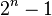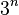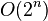# God's algorithm facts for kids

Kids Encyclopedia Facts

God's algorithm is a notion originating in discussions of ways to solve the Rubik's Cube puzzle, but which can also be applied to other combinatorial puzzles and mathematical games. It refers to any algorithm which produces a solution having the fewest possible moves, the idea being that only an omniscient being would know an optimal step from any given configuration.

## Scope

### Definition

The notion applies to puzzles that can assume a finite number of "configurations", with a relatively small, well-defined arsenal of "moves" that may be applicable to configurations and then lead to a new configuration. Solving the puzzle means to reach a designated "final configuration", a singular configuration, or one of a collection of configurations. To solve the puzzle a sequence of moves is applied, starting from some arbitrary initial configuration.

### Solution

An algorithm can be considered to solve such a puzzle if it takes as input an arbitrary initial configuration and produces as output a sequence of moves leading to a final configuration (if the puzzle is solvable from that initial configuration, otherwise it signals the impossibility of a solution). A solution is optimal if the sequence of moves is as short as possible. This count is known as God's number, or, more formally, the minimax value. God's algorithm, then, for a given puzzle, is an algorithm that solves the puzzle and produces only optimal solutions.

Some writers, such as David Joyner, consider that for an algorithm to be properly referred to as "God's algorithm", it should also be practical, meaning that the algorithm does not require extraordinary amounts of memory or time. For example, using a giant lookup table indexed by initial configurations would allow solutions to be found very quickly, but would require an extraordinary amount of memory.

Instead of asking for a full solution, one can equivalently ask for a single move from an initial but not final configuration, where the move is the first of some optimal solution. An algorithm for the single-move version of the problem can be turned into an algorithm for the original problem by invoking it repeatedly while applying each move reported to the present configuration, until a final one is reached. Conversely, any algorithm for the original problem can be turned into an algorithm for the single-move version by truncating its output to its first move.

## Examples

Well-known puzzles fitting this description are mechanical puzzles like Rubik's Cube, Towers of Hanoi, and the 15 puzzle. The one-person game of peg solitaire is also covered, as well as many logic puzzles, such as the missionaries and cannibals problem. These have in common that they can be modeled mathematically as a directed graph, in which the configurations are the vertices, and the moves the arcs.

### Mechanical puzzles

#### n-Puzzles

The Fifteen puzzle can be solved in 80 single-tile moves or 43 multi-tile moves in the worst case. For its generalization the n-puzzle, the problem of finding an optimal solution is NP-hard. Therefore, whether a practical God's algorithm for this problem exists remains unknown, but appears unlikely.

#### Towers of Hanoi

For the Towers of Hanoi puzzle, a God's algorithm is known for any given number of disks. The number of moves is exponential in the number of disks ($2^n-1$).

#### Rubik's Cube

An algorithm to determine the minimum number of moves to solve Rubik's Cube was published in 1997 by Richard Korf. While it had been known since 1995 that 20 was a lower bound on the number of moves for the solution in the worst case, it was proven in 2010 through extensive computer calculations that no configuration requires more than 20 moves. Thus 20 is a sharp upper bound on the length of optimal solutions. Mathematician David Singmaster had "rashly conjectured" this number to be 20 in 1980.

## Unsolved games

Some well known games with a very limited set of simple well-defined rules and moves have nevertheless never had their God's algorithm for a winning strategy determined. Examples are the board games chess and Go. Both these games have a rapidly increasing number of positions with each move. The total number of all possible positions, approximately 10154 for chess and 10180 (on a 19×19 board) for Go, is much too large to allow a brute force solution with current computing technology (compare the now solved, with great difficulty, Rubik's Cube at only about 4.3×1019 positions). Consequently, a brute force determination of God's algorithm for these games is not possible. While chess computers have been built that are capable of beating even the best human players, they do not calculate the game all the way to the end. Deep Blue, for instance, searched only 11 moves ahead (counting a move by each player as two moves), reducing the search space to only 1017. After this, it assessed each position for advantage according to rules derived from human play and experience.

Even this strategy is not possible with Go. Besides having hugely more positions to evaluate, no one so far has successfully constructed a set of simple rules for evaluating the strength of a Go position as has been done for chess. Evaluation algorithms are prone to make elementary mistakes so even for a limited look ahead with the goal limited to finding the strongest interim position, a God's algorithm has not been possible for Go.

On the other hand, draughts (checkers), with superficial similarities to chess, has long been suspected of being "played out" by its expert practitioners. In 2007 Schaeffer et al. proved this to be so by calculating a database of all positions with ten or fewer pieces. Thus Schaeffer has a God's algorithm for all end games of draughts and used this to prove that all perfectly played games of draughts will end in a draw. However, draughts with only 5×1020 positions and even fewer, 3.9×1013, in Schaeffer's database, is a much easier problem to crack and is of the same order as Rubik's cube.

The magnitude of the set of positions of a puzzle does not entirely determine whether a God's algorithm is possible. The already solved Tower of Hanoi puzzle can have an arbitrary number of pieces, and the number of positions increases exponentially as$3^n$. Nevertheless, the solution algorithm is applicable to any size problem, and since the algorithm running time is$O(2^n)$ the problem is NP-hard.God's algorithm Facts for Kids. Kiddle Encyclopedia.